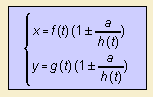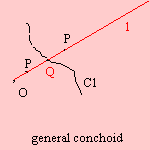# (generalized) conchoid

## mainwith h(t) = √(f2(t)+g2(t))Given a curve C1(f(t), g(t)) and a fixed point O(0,0). Draw lines l through O that intersect C1 in Q. Then the conchoid is defined as the collection of points P (on l) for which PQ is equal to a constant a.

This conchoid is a generalization of the 1st curve that was named as a conchoid, the (simple) conchoid that is derived from the straight line.

In the following table some conchoids have been collected:
 C1 O conchoid of C1 Archimedes' spiral center Archimedes' spiral circle on C1 limaçon  When the constant a is equal to the diameter of C1 the cardioid appears. not on C1 conchoid of a circle curve with polar equation r = f(φ) the origin curve with polar equation r = f(φ) + a rose center botanic curve straight line (simple) conchoid

The conchoid of Dürer does not belong to this family, it is another variation on the conchoid.